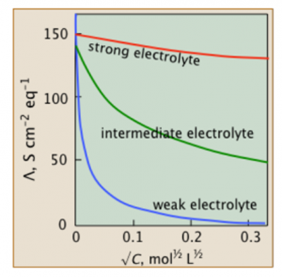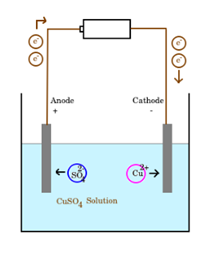# Molar Conductivity

## What is Molar Conductivity?

Molar conductivity is the conductance property of a solution containing one mole of the electrolyte or it is a function of the ionic strength of a solution or the concentration of salt. It is therefore not a constant.

In other words, molar conductivity can also be defined as the conducting power of all the ions that are formed by dissolving a mole of electrolyte in a solution. Molar conductivity is the property of an electrolyte solution that is mainly used in determining the efficiency of a given electrolyte in conducting electricity in a solution. It is therefore not a constant.

### Molar Conductivity Formula

The following expression is used to mathematically represent molar conductivity.

Λm = K / C

Where K is the specific conductivity and c is the concentration in mole per litre.

In general, the molar conductivity of an electrolytic solution is the conductance of the volume of the solution containing a unit mole of electrolyte that is placed between two electrodes of unit area cross-section or at a distance of one-centimeter apart.

The unit of molar conductivity is S⋅m2⋅mol-1.

## Variation of Molar Conductivity with Concentration

The molar conductivity of both weak and strong electrolytes increases with a decrease in concentration or dilution. We know that the molar conductivity is the conductivity offered by one mole of ions. Even after dilution, we are still considering the same unit mole of ions. But however, the increased dilution results in the dissociation of more electrolytes into ions and effectively increasing the number of active ions in the solution. These active ions impart more conductivity.The above plot explains that for strong electrolytes, the molar conductivity is increasing slowly with the dilution. If EO m is the limiting molar conductivity (the molar conductivity at zero concentration), then the general equation for the plot of the strong electrolyte can be expressed with the help of Kohlrausch’s law;

Λm = Eom – A √ c

Where A is the slope of the plot. It depends on the type of electrolyte at a given temperature for a given solvent.

For weak electrolytes, however, the molar conductivity shoots at lower concentrations. Conversely, such electrolytes have lower molar conductivity at higher concentrations due to a reduced degree of dissociation.

In the case of specific conductivity, it is seen that the conductivity increases as the concentration of the electrolyte increases. The specific conductivity depends on the number of ions present in the unit volume of the solution. On dilution, the dissociation increases, causing the current-carrying ions to increase in the solution. But due to dilution, the number of ions present in a unit volume of the solution decrease. This causes a reduction in conductivity.

For strong electrolytes, the increase in concentration produces a sharp increase in conductivity. How ever, at lower concentration weak electrolytes posses very low values of specific conductivity and the value gradually increases as the concentration is increased. This is due to the increase in the number of active ions in the solution due to concentration.

## Specific Conductivity

The extent to which a given sample of electrolytic solution can conduct an electric current is called its conductance. Conversely, the extent to which the given sample of electrolytic solution can resist the flow of electric current is called its resistance. Of course, the conductance is the reciprocal of resistance of that particular electrolytic solution. The SI unit of conductance is S (Siemens).

The conductivity or specific conductivity is the measure of the ability of an electrolytic solution to conduct electricity. The SI unit of specific conductivity is Siemens per meter, better represented as S/m.

G = 1 / R

We have R = ρ l / A

G = 1 / ρ X A / l

G = k A / l

Where ρ is the specific resistivity and k is the specific conductivity. This is the reciprocal of resistivity.

K 1 / ρ = C / R

In general, we define the specific conductivity of an electrolytic solution at any given concentration as the conductance of one unit volume of solution that is placed between two platinum electrodes with the unit area of cross-section.

The specific conductivity of an electrolytic solution depends on the following factors:

● Nature and the concentration of the electrolyte in the solution.

● The size of the ions within the solution.

● Solvent nature and its viscosity.

● The temperature.

Different electrolytic solutions have different concentrations and therefore contain different numbers of ions. Due to this, specific conductivity is not a suitable quantity to compare the conductance of different electrolytic solutions. For this, molar conductivity is introduced.

## Electrolytic Conduction

Electrolytes conduct electricity due to the presence of free ions contained in it. It is similar to the case of metallic conductors, where free electrons favour the conduction of electricity. The electrolytic conduction is explained based on the Arrhenius equation or theory.

We know that electrolytic solutions that are made from the dissolution of certain salts. The salts need not be always ionic in nature. The only criteria are that the compound should be composed of oppositely charged ions.

Arrhenius theory explains that when a neutral electrolyte is dissolved in water, the electrolyte molecules are split into two differently charged ions. The positively charged one is called the cation and the negatively charged one is called an anion. The theory assumes that ions are already there in the electrolyte, but however, bound together by electrostatic force and hence they are neutral. But once dissolved, the ions dissociate from their bound form.

AB → A + B

However, there will be a recombination of ions also take place. Hence, the actual reaction occurring within the electrolytic solution is a reversible reaction. It is represented below.

AB ⇄ A+ + B

The charged particles are able to move freely through the solution. The positive ions or cations are able to move towards a negative electrode or cathode to get itself reduced. At the same time, the negative ions or anions are able to move towards the positive electrode or anode to get itself oxidized. This movement of charged particles called migration imparts electric conduction.

Let us look at an example. Given below is an electrolytic cell containing a copper sulfate solution.The free electrons are drifted through the metallic conductor due to the potential difference created by the cell. These electrons reach the cathode making it negative. Due to this polarity, the copper ions are attracted to it. These copper ions consume the electrons at the electrodes disposal and reduced to copper metal. The consumed electrons are replenished at the anode when sulfate ions are oxidized. The oxidization leaves sets of two electrons behind. These electrons are taken back to the cell.

## Problems Related To Molar Conductivity

1. calculate the molar conductivity of the KCl solution?

Given:

Molarity (M) = 0.30M

Conductivity at 298 K (k) = 0.023 S cm

Solution:

Molar conductivity = (1000 × k) /M

= (1000 × 0.023) / 0.30

= 76.66 cm² mol⁻¹

Molar conductivity of the KCl solution is 76.66 cm² mol⁻¹.

2. COnductivity of 0.02 M solution of KCl at 298 K is 0.0248 S cm– . Calculate the molar conductivity?

Given:

Molarity (M) = 0.20M

Conductivity at 298 K (k) = 0.0248 S cm

Solution:

Molar conductivity = (1000 × k) /M

= (1000 × 0.0248) / 0.20

= 124‬ cm² mol⁻¹

Molar conductivity of the KCl solution is 124‬ cm² mol⁻¹

3.  Molar conductance values at infinite dilution of Na+ and Cl- ions are 51.12 × 10-4 Sm2mol-1 and 73.54× 10-4 Sm2mol-1 respectively. Calculate the total molar conductance of NaCl?

Solution:

Molar conductance at infinite dilution

λ+Na = 51.12×10Sm2mol−1
λ+Cl = 73.54×10Sm2mol−1

Molar conductance of NaCl = λ+Na + λ+Cl

= 51.12×104  + 73.54×104

= 124.66 ×10 Sm2mol−1

Molar conductance of NaCl is 124.66 ×10 Sm2mol−1

1. Give the relationship between specific conductance and molar conductance?

Answer: When the molarity of the solution is multiplied by the molar conductance of the solution it gives specific conductance.

2. What is the relationship between the molar conductance and the dilution? how does it vary with the conductance of the solution?